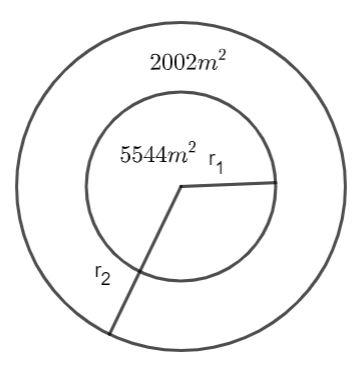QuestionAnswers

# The area of a circular garden is 5544 ${m^2}$. Outside this garden, a path of uniform width is laid all around. The area of the path is 2002 ${m^2}$. Find:(i). the radius of the circular garden.(ii). the width of the path.Verified
113.4K+ Views
Hint: The area of a circle of radius r is $\pi {r^2}$. Use this formula to find the radius of the circular garden and the circle including the path. Then, the difference between these radii is the width of the path.

The length between the center of the circle and the points on the circle is fixed and is called the radius of the circle.
The area of the circle of radius r is given as follows:
$A = \pi {r^2}............(1)$The area of the circular garden is given to be 5544 ${m^2}$, then, by using equation (1), we can find the radius of the circular garden.
$5544 = \dfrac{{22}}{7}{r_1}^2$
Solving for ${r_1}$, we have:
${r_1}^2 = \dfrac{7}{{22}} \times 5544$
${r_1}^2 = 1764$
We know that 42 squares is 1764.
${r_1} = 42m...........(2)$
Hence, the radius of the circular garden is 42 m.
The area of the path is given as 2002 ${m^2}$, the total area of the bigger circle is the sum of the area of the path and the area of the garden.
${A_2} = 5544 + 2002$
${A_2} = 7546c{m^2}$
Using equation (1), we can find the radius of the bigger circle.
$7546 = \dfrac{{22}}{7}r_2^2$
Solving for ${r_2}$, we have:
$r_2^2 = \dfrac{7}{{22}} \times 7546$
$r_2^2 = 2401$
We know that square of 49 is 2401.
${r_2} = 49m..........(3)$
The width of the path is the difference between the equations (2) and (3).
Width of the path = ${r_2} - {r_1}$
Width of the path = 49 – 42
Width of the path = 7 m
Hence, the width of the path is 7 m.

Note: When taking the square root, we get both positive and negative values but the only positive value is feasible. Do not find the width of the path by equating 2002 to $\pi {r^2}$, it will result in the wrong answer.